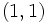# Subgroup structure of general linear group:GL(2,3)

View subgroup structure of particular groups | View other specific information about general linear group:GL(2,3)

This article discusses the subgroup structure of the general linear group GL(2,3).

Here is the list:

1. The trivial group. (1)
2. The center, which is a two-element subgroup isomorphic to a cyclic group of order two. It comprises the identity element and its negative. (1)
3. The conjugates to the two-element subgroup generated by$\begin{pmatrix}1 & 0 \\ 0 & -1 \\\end{pmatrix}$. (12)
4. Subgroups of order three, isomorphic to the cyclic group of order three, all conjugate to the subgroup$\langle \begin{pmatrix}1 & 1 \\ 0 & 1 \\\end{pmatrix}\rangle$. (4)
5. Subgroups of order four, isomorphic to the cyclic group of order four all conjugate to the subgroup$\langle \begin{pmatrix} 0 & 1 \\ 2 & 0 \\\end{pmatrix}\rangle$. (3)
6. Subgroups of order four, isomorphic to Klein four-group, all conjugate to the subgroup of diagonal matrices. (6)
7. Subgroups of order six, isomorphic to the cyclic group of order six, all conjugate to the subgroup$\langle \begin{pmatrix}2 & 2 \\ 0 & 2 \\\end{pmatrix}\rangle$. (4)
8. Subgroups of order six, isomorphic to the symmetric group of degree three. These are all automorphic to each other, but they come in two conjugacy classes of size four each. An example is$\langle \begin{pmatrix}1 & 1 \\ 0 & 1 \\\end{pmatrix}, \begin{pmatrix}1 & 0 \\ 0 & -1 \\\end{pmatrix} \rangle$.(8)
9. A subgroup of order eight, isomorphic to the quaternion group. (1)
10. Subgroups of order eight, isomorphic to dihedral group:D8. These are all conjugate subgroups. An example is the the orthogonal group$O(2,3)$, i.e., the subgroup$\langle \begin{pmatrix}0 & 1 \\ 1 & 0 \\\end{pmatrix}, \begin{pmatrix}1 & 0 \\ 0 & -1 \\\end{pmatrix}\rangle$.(3)
11. Subgroups of order eight, isomorphic to cyclic group:Z8. These are all conjugate, and an example is$\langle \begin{pmatrix} 1 & 1 \\ 2 & 1 \\\end{pmatrix}\rangle$.(3)
12. Subgroups of order twelve, isomorphic to dihedral group:D12. These are all conjugate to each other. One example is$\langle \begin{pmatrix}1 & 1 \\ 0 & 1 \\\end{pmatrix}, \begin{pmatrix}1 & 0 \\ 0 & -1 \\\end{pmatrix}, \begin{pmatrix}-1 & 0 \\ 0 & 1 \\\end{pmatrix} \rangle$. (4)
13. Subgroups of order sixteen, isomorphic to semidihedral group:SD16. These are all conjugate subgroups and are the 2-Sylow subgroups. (3)
14. A unique subgroup of order$24$, namely the special linear group:SL(2,3). (1)
15. The whole group. (1)

## Tables for quick information

### Table classifying isomorphism types of subgroups

Group name GAP ID Occurrences as subgroup Conjugacy classes of occurrence as subgroup Occurrences as normal subgroup Occurrences as characteristic subgroup
Trivial group$(1,1)$ 1 1 1 1
Cyclic group:Z2$(2,1)$ 13 2 1 1
Cyclic group:Z3$(3,1)$ 4 1 0 0
Cyclic group:Z4$(4,1)$ 3 1 0 0
Klein four-group$(4,2)$ 6 1 0 0
Cyclic group:Z6$(6,2)$ 4 1 0 0
Symmetric group:S3$(6,1)$ 8 2 0 0
Quaternion group$(8,4)$ 1 1 1 1
Dihedral group:D8$(8,3)$ 3 1 0 0
Cyclic group:Z8$(8,1)$ 3 1 0 0
Dihedral group:D12$(12,4)$ 4 1 0 0
Semidihedral group:SD16$(16,8)$ 3 1 0 0
Special linear group:SL(2,3)$(24,3)$ 1 1 1 1
General linear group:GL(2,3)$(48,29)$ 1 1 1 1
Total -- 55 16 5 5

### Table listing number of subgroups by order

Group order Occurrences as subgroup Conjugacy classes of occurrence as subgroup Occurrences as normal subgroup Occurrences as characteristic subgroup$1$ 1 1 1 1$2$ 13 2 1 1$3$ 4 1 0 0$4$ 9 2 0 0$6$ 12 3 0 0$8$ 7 3 1 1$12$ 4 1 0 0$16$ 3 1 0 0$24$ 1 1 1 1$48$ 1 1 1 1
Total 55 16 5 5